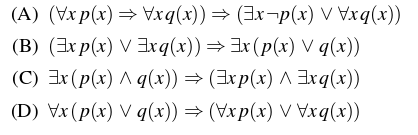Skip to content
Related Articles
GATE | GATE-CS-2016 (Set 2) | Question 37
• Difficulty Level : Hard
• Last Updated : 13 Feb, 2016

Which one of the following well-formed formulae in predicate calculus is NOT valid?(A) A
(B) B
(C) C
(D) D

Answer: (D)

Explanation:

```Suppose if there are two statements P and Q,
P=>Q = ~PvQ i.e.
The only situation where implication fails is (=>) when P is true and Q is false.
i.e. A truth statement can't imply a false statement.
So, for these type of questions it will be better to take option and
check for some arbitrary condition
By looking options, we are pretty sure that A,B are correct
Suppose X is any number and statement is P(x) = X is a prime number
Q(x) = X is a non-prime number
If we look at option D,
Before Implication :- For all x, x is either prime or non-prime which is true
After Implication  :-   For all x, x is prime or for all x, x is non-prime which is
obviously false i.e. here, truth statement implies a false statement which is not valid.

If we carefully look at option C,
There exists a number x, which is both prime and non-prime which is false
and a false statement can imply either true or false. So option (C) is correct
So Answer is Option (D)
```

This explanation has been contributed by Anil Saikrishna.

Quiz of this Question

Attention reader! Don’t stop learning now. Learn all GATE CS concepts with Free Live Classes on our youtube channel.

My Personal Notes arrow_drop_up